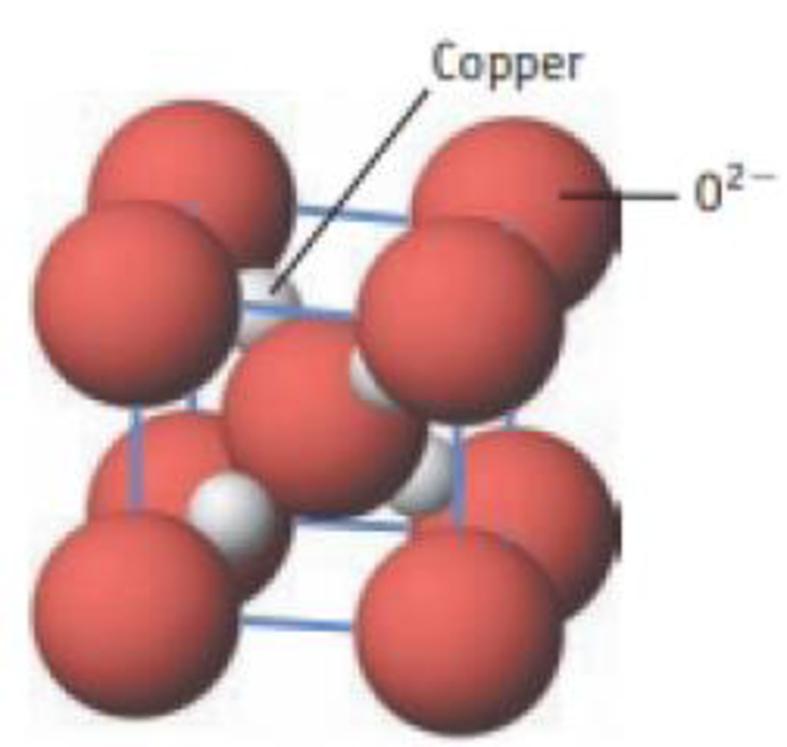Chapter 12, Problem 7PS

Chapter
Section
Textbook Problem

Cuprite is a semiconductor. Oxide ions are at the cube comers and in the cube center. Copper ions are wholly within the unit cell. (a) What is the formula of cuprite? (b) What is the oxidation number of copper?Unit cell for cuprite

(a)

Interpretation Introduction

Interpretation:

The formula for cuprite has to be identified.

Concept introduction:

Molecular formula shows that the actual number of atoms of each element that are present in a molecule of the compound.

Explanation

According to the picture, there are eight O2- ion at eight corners therefore and one O2- ion in the center, therefore totally two O2- ion in the unit cell. There are four Cu atom is present in the inner tetrahedral holes.

Number of O2-atoms per unit=(8corneratoms×1/8)Number of O2-atoms per unit=

(b)

Interpretation Introduction

Interpretation:

The oxidation number of copper has to be identified.

Concept introduction:

• An oxidizing agent gains the electrons and is reduced in a chemical reaction and it is electron acceptor.
• A reducing agent loses electrons and is oxidized in a chemical reaction and it is electron donor.
• The oxidation state is called as oxidation number, which describes degree of oxidation (loss of electrons) of an atom in a chemical compound. Theoretically, the oxidation state is positive, negative or zero.

Still sussing out bartleby?

Check out a sample textbook solution.

See a sample solution

The Solution to Your Study Problems

Bartleby provides explanations to thousands of textbook problems written by our experts, many with advanced degrees!

Get Started

How do eating disorders affect health?

Understanding Nutrition (MindTap Course List)

What is the structure and function of a chloroplast?

Biology: The Dynamic Science (MindTap Course List)

What are genomes?

Human Heredity: Principles and Issues (MindTap Course List)